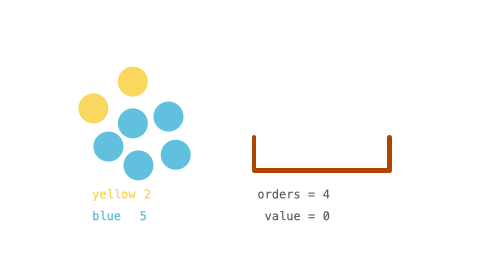# 销售价值减少的颜色球

## 1648. 销售价值减少的颜色球 (Medium)```输入：inventory = [2,5], orders = 4

```

```输入：inventory = [3,5], orders = 6

```

```输入：inventory = [2,8,4,10,6], orders = 20

```

```输入：inventory = , orders = 1000000000

```

• `1 <= inventory.length <= 105`
• `1 <= inventory[i] <= 109`
• `1 <= orders <= min(sum(inventory[i]), 109)`

[贪心算法] [排序] [数学]